CalculusShowing results 21 to 30 of 52, on page 3 of 6 Differentiation/Differentals Integration/Integrals Limits Sequences and Series
 DESCRIPTION EQUATION Derivative of Hyperbolic Tangent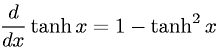Derivative of Inverse Cosecant (Arccosecant)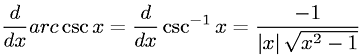Derivative of Inverse Cosine (Arccosine)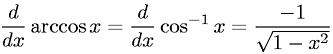Derivative of Inverse Cotangent (Arccotangent)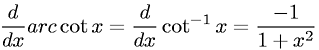Derivative of Inverse Secant (Arcsecant)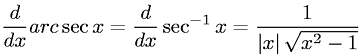Derivative of Inverse Sine (Arcsine)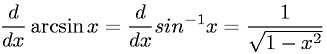Derivative of Inverse Tangent (Arctangent)Derivative of Secant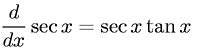Derivative of SineDerivative of Tangent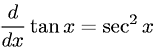Go to page: 1  2  3  4  5  6